Search by Topic

Filter by: Content type:
Age range:
Challenge level:

There are 199 NRICH Mathematical resources connected to Addition & subtraction, you may find related items under Calculations and Numerical Methods.

Broad Topics > Calculations and Numerical Methods > Addition & subtractionAge 5 to 11 Challenge Level:

Try out this number trick. What happens with different starting numbers? What do you notice?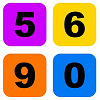Always, Sometimes or Never? KS1

Age 5 to 7 Challenge Level:

Are these statements relating to calculation and properties of shapes always true, sometimes true or never true?Subtraction Surprise

Age 7 to 14 Challenge Level:

Try out some calculations. Are you surprised by the results?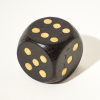Double or Halve?

Age 5 to 7 Challenge Level:

Throw the dice and decide whether to double or halve the number. Will you be the first to reach the target?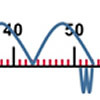Five Steps to 50

Age 5 to 7 Challenge Level:

Use five steps to count forwards or backwards in 1s or 10s to get to 50. What strategies did you use?Take Three Numbers

Age 7 to 11 Challenge Level:

What happens when you add three numbers together? Will your answer be odd or even? How do you know?Dotty Six

Age 5 to 11 Challenge Level:

Dotty Six is a simple dice game that you can adapt in many ways.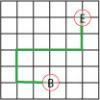Journeys in Numberland

Age 7 to 11 Challenge Level:

Tom and Ben visited Numberland. Use the maps to work out the number of points each of their routes scores.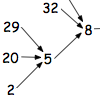Age 7 to 11 Challenge Level:

What happens when you add the digits of a number then multiply the result by 2 and you keep doing this? You could try for different numbers and different rules.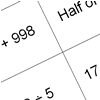How Do You Do It?

Age 7 to 11 Challenge Level:

This group activity will encourage you to share calculation strategies and to think about which strategy might be the most efficient.Even and Odd

Age 5 to 7 Challenge Level:

This activity is best done with a whole class or in a large group. Can you match the cards? What happens when you add pairs of the numbers together?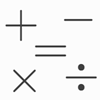Which Symbol?

Age 7 to 11 Challenge Level:

Choose a symbol to put into the number sentence.Nice or Nasty

Age 7 to 14 Challenge Level:

There are nasty versions of this dice game but we'll start with the nice ones...Countdown

Age 7 to 14 Challenge Level:

Here is a chance to play a version of the classic Countdown Game.Magic Vs

Age 7 to 11 Challenge Level:

Can you put the numbers 1-5 in the V shape so that both 'arms' have the same total?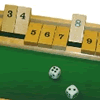Shut the Box

Age 5 to 7 Challenge Level:

An old game but lots of arithmetic!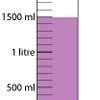Oh! Harry!

Age 7 to 11 Challenge Level:

A group of children are using measuring cylinders but they lose the labels. Can you help relabel them?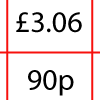How Much Did it Cost?

Age 7 to 11 Challenge Level:

Use your logical-thinking skills to deduce how much Dan's crisps and ice-cream cost altogether.Sea Level

Age 7 to 11 Challenge Level:

The picture shows a lighthouse and many underwater creatures. If you know the markings on the lighthouse are 1m apart, can you work out the distances between some of the different creatures?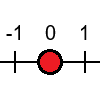Tug Harder!

Age 7 to 11 Challenge Level:

In this game, you can add, subtract, multiply or divide the numbers on the dice. Which will you do so that you get to the end of the number line first?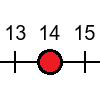Tug of War

Age 5 to 7 Challenge Level:

Can you use the numbers on the dice to reach your end of the number line before your partner beats you?First Connect Three

Age 7 to 14 Challenge Level:

Add or subtract the two numbers on the spinners and try to complete a row of three. Are there some numbers that are good to aim for?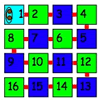Caterpillars

Age 5 to 7 Challenge Level:

These caterpillars have 16 parts. What different shapes do they make if each part lies in the small squares of a 4 by 4 square?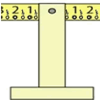Balance of Halves

Age 7 to 11 Challenge Level:

Investigate this balance which is marked in halves. If you had a weight on the left-hand 7, where could you hang two weights on the right to make it balance?Making Longer, Making Shorter

Age 5 to 7 Challenge Level:

Ahmed is making rods using different numbers of cubes. Which rod is twice the length of his first rod?Domino Pick

Age 5 to 7 Challenge Level:

Are these domino games fair? Can you explain why or why not?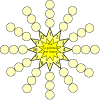The Twelve Pointed Star Game

Age 7 to 11 Challenge Level:

Have a go at this game which involves throwing two dice and adding their totals. Where should you place your counters to be more likely to win?Diagonal Sums

Age 7 to 11 Challenge Level:

In this 100 square, look at the green square which contains the numbers 2, 3, 12 and 13. What is the sum of the numbers that are diagonally opposite each other? What do you notice?Number Differences

Age 7 to 11 Challenge Level:

Place the numbers from 1 to 9 in the squares below so that the difference between joined squares is odd. How many different ways can you do this?Ring a Ring of Numbers

Age 5 to 7 Challenge Level:

Choose four of the numbers from 1 to 9 to put in the squares so that the differences between joined squares are odd.Carrying Cards

Age 7 to 11 Challenge Level:

These sixteen children are standing in four lines of four, one behind the other. They are each holding a card with a number on it. Can you work out the missing numbers?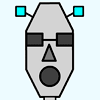Robot Monsters

Age 5 to 7 Challenge Level:

Use these head, body and leg pieces to make Robot Monsters which are different heights.A Mixed-up Clock

Age 7 to 11 Challenge Level:

There is a clock-face where the numbers have become all mixed up. Can you find out where all the numbers have got to from these ten statements?The Deca Tree

Age 7 to 11 Challenge Level:

Find out what a Deca Tree is and then work out how many leaves there will be after the woodcutter has cut off a trunk, a branch, a twig and a leaf.A Square of Numbers

Age 7 to 11 Challenge Level:

Can you put the numbers 1 to 8 into the circles so that the four calculations are correct?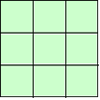1, 2, 3 Magic Square

Age 7 to 11 Challenge Level:

Arrange three 1s, three 2s and three 3s in this square so that every row, column and diagonal adds to the same total.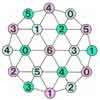Totality

Age 5 to 11 Challenge Level:

This is an adding game for two players.Cubes Within Cubes

Age 7 to 14 Challenge Level:

We start with one yellow cube and build around it to make a 3x3x3 cube with red cubes. Then we build around that red cube with blue cubes and so on. How many cubes of each colour have we used?Two Primes Make One Square

Age 7 to 11 Challenge Level:

Can you make square numbers by adding two prime numbers together?One Wasn't Square

Age 7 to 11 Challenge Level:

Mrs Morgan, the class's teacher, pinned numbers onto the backs of three children. Use the information to find out what the three numbers were.The Tomato and the Bean

Age 5 to 7 Challenge Level:

At the beginning of May Tom put his tomato plant outside. On the same day he sowed a bean in another pot. When will the two be the same height?Abundant Numbers

Age 7 to 11 Challenge Level:

48 is called an abundant number because it is less than the sum of its factors (without itself). Can you find some more abundant numbers?Zios and Zepts

Age 7 to 11 Challenge Level:

On the planet Vuv there are two sorts of creatures. The Zios have 3 legs and the Zepts have 7 legs. The great planetary explorer Nico counted 52 legs. How many Zios and how many Zepts were there?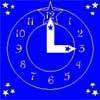Watch the Clock

Age 7 to 11 Challenge Level:

During the third hour after midnight the hands on a clock point in the same direction (so one hand is over the top of the other). At what time, to the nearest second, does this happen?What's in a Name?

Age 5 to 7 Challenge Level:

What do you notice about these squares of numbers? What is the same? What is different?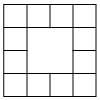Prison Cells

Age 7 to 11 Challenge Level:

There are 78 prisoners in a square cell block of twelve cells. The clever prison warder arranged them so there were 25 along each wall of the prison block. How did he do it?Neighbours

Age 7 to 11 Challenge Level:

In a square in which the houses are evenly spaced, numbers 3 and 10 are opposite each other. What is the smallest and what is the largest possible number of houses in the square?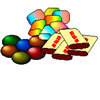The Puzzling Sweet Shop

Age 7 to 11 Challenge Level:

There were chews for 2p, mini eggs for 3p, Chocko bars for 5p and lollypops for 7p in the sweet shop. What could each of the children buy with their money?The Amazing Splitting Plant

Age 5 to 7 Challenge Level:

Can you work out how many flowers there will be on the Amazing Splitting Plant after it has been growing for six weeks?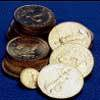Five Coins

Age 7 to 11 Challenge Level:

Ben has five coins in his pocket. How much money might he have?